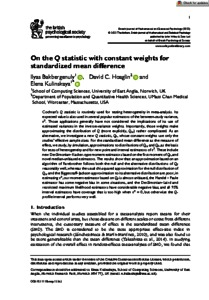# On the Q statistic with constant weights for standardized mean difference

Bakbergenuly, Ilyas, Hoaglin, David C. and Kulinskaya, Elena (2022) On the Q statistic with constant weights for standardized mean difference. British Journal of Mathematical and Statistical Psychology, 75 (3). pp. 444-465. ISSN 0007-1102Preview PDF (Published_Version) - Published Version Available under License Creative Commons Attribution. Download (564kB) | Preview

## Abstract

Cochran's Q statistic is routinely used for testing heterogeneity in meta-analysis. Its expected value is also used in several popular estimators of the between-study variance, (Formula presented.). Those applications generally have not considered the implications of its use of estimated variances in the inverse-variance weights. Importantly, those weights make approximating the distribution of Q (more explicitly, (Formula presented.)) rather complicated. As an alternative, we investigate a new Q statistic, (Formula presented.), whose constant weights use only the studies' effective sample sizes. For the standardized mean difference as the measure of effect, we study, by simulation, approximations to distributions of (Formula presented.) and (Formula presented.), as the basis for tests of heterogeneity and for new point and interval estimators of (Formula presented.). These include new DerSimonian–Kacker-type moment estimators based on the first moment of (Formula presented.), and novel median-unbiased estimators. The results show that: an approximation based on an algorithm of Farebrother follows both the null and the alternative distributions of (Formula presented.) reasonably well, whereas the usual chi-squared approximation for the null distribution of (Formula presented.) and the Biggerstaff–Jackson approximation to its alternative distribution are poor; in estimating (Formula presented.), our moment estimator based on (Formula presented.) is almost unbiased, the Mandel – Paule estimator has some negative bias in some situations, and the DerSimonian–Laird and restricted maximum likelihood estimators have considerable negative bias; and all 95% interval estimators have coverage that is too high when (Formula presented.), but otherwise the Q-profile interval performs very well.

Item Type: Article Funding Information: The work by E. Kulinskaya was supported by the Economic and Social Research Council [grant number ES/L011859/1]. statistics and probability,arts and humanities (miscellaneous),psychology(all) ,/dk/atira/pure/subjectarea/asjc/2600/2613 Faculty of Science > School of Computing Sciences LivePure Connector 16 Feb 2022 14:30 21 Apr 2023 01:24 https://ueaeprints.uea.ac.uk/id/eprint/83530 10.1111/bmsp.12263View Item